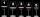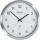# Fraction calculator

The calculator performs basic and advanced operations with fractions, expressions with fractions combined with integers, decimals, and mixed numbers. It also shows detailed step-by-step information about the fraction calculation procedure. Solve problems with two, three, or more fractions and numbers in one expression.

## Result:

### 9/22 : 12/11 = 3/8 = 0.375

Spelled result in words is three eighths.

### How do you solve fractions step by step?

1. Divide: 9/22 : 12/11 = 9/22 · 11/12 = 9 · 11/22 · 12 = 99/264 = 33 · 3 /33 · 8 = 3/8
Dividing two fractions is the same as multiplying the first fraction by the reciprocal value of the second fraction. The first sub-step is to find the reciprocal (reverse the numerator and denominator, reciprocal of 12/11 is 11/12) of the second fraction. Next, multiply the two numerators. Then, multiply the two denominators. In the following intermediate step, cancel by a common factor of 33 gives 3/8.
In other words - nine twenty-seconds divided by twelve elevenths = three eighths.

#### Rules for expressions with fractions:

Fractions - use the slash “/” between the numerator and denominator, i.e., for five-hundredths, enter 5/100. If you are using mixed numbers, be sure to leave a single space between the whole and fraction part.
The slash separates the numerator (number above a fraction line) and denominator (number below).

Mixed numerals (mixed fractions or mixed numbers) write as non-zero integer separated by one space and fraction i.e., 1 2/3 (having the same sign). An example of a negative mixed fraction: -5 1/2.
Because slash is both signs for fraction line and division, we recommended use colon (:) as the operator of division fractions i.e., 1/2 : 3.

Decimals (decimal numbers) enter with a decimal point . and they are automatically converted to fractions - i.e. 1.45.

The colon : and slash / is the symbol of division. Can be used to divide mixed numbers 1 2/3 : 4 3/8 or can be used for write complex fractions i.e. 1/2 : 1/3.
An asterisk * or × is the symbol for multiplication.
Plus + is addition, minus sign - is subtraction and ()[] is mathematical parentheses.
The exponentiation/power symbol is ^ - for example: (7/8-4/5)^2 = (7/8-4/5)2

#### Examples:

subtracting fractions: 2/3 - 1/2
multiplying fractions: 7/8 * 3/9
dividing Fractions: 1/2 : 3/4
exponentiation of fraction: 3/5^3
fractional exponents: 16 ^ 1/2
adding fractions and mixed numbers: 8/5 + 6 2/7
dividing integer and fraction: 5 ÷ 1/2
complex fractions: 5/8 : 2 2/3
decimal to fraction: 0.625
Fraction to Decimal: 1/4
Fraction to Percent: 1/8 %
comparing fractions: 1/4 2/3
multiplying a fraction by a whole number: 6 * 3/4
square root of a fraction: sqrt(1/16)
reducing or simplifying the fraction (simplification) - dividing the numerator and denominator of a fraction by the same non-zero number - equivalent fraction: 4/22
expression with brackets: 1/3 * (1/2 - 3 3/8)
compound fraction: 3/4 of 5/7
fractions multiple: 2/3 of 3/5
divide to find the quotient: 3/5 ÷ 2/3

The calculator follows well-known rules for order of operations. The most common mnemonics for remembering this order of operations are:
PEMDAS - Parentheses, Exponents, Multiplication, Division, Addition, Subtraction.
BEDMAS - Brackets, Exponents, Division, Multiplication, Addition, Subtraction
BODMAS - Brackets, Of or Order, Division, Multiplication, Addition, Subtraction.
GEMDAS - Grouping Symbols - brackets (){}, Exponents, Multiplication, Division, Addition, Subtraction.
Be careful, always do multiplication and division before addition and subtraction. Some operators (+ and -) and (* and /) has the same priority and then must evaluate from left to right.

## Fractions in word problems:

• Ten fractionsWrite ten fractions between 1/3 and 2/3
• ExpressionsLet k represent an unknown number, express the following expressions: 1. The sum of the number n and two 2. The quotient of the numbers n and nine 3. Twice the number n 4. The difference between nine and the number n 5. Nine less than the number n
• Quotient 3If the quotient of 8/13 and 2 is subtracted from the product of 1 3/4 and 8/21, what is the difference?
• The quotientThe quotient of g and 55 is the same as 279. What is g?
• Find the 11Find the quotient of 229.12 and 12.32
• DivideDivide the number 72 in the ratio 7: 2 and calculate the ratio of the numbers found in this order and write down as decimal.
• CupsWe have three cups. In the cups we had fluid and boredom we started to shed. 1 We shed one-third of the fluid from the second glass into the first and third. 2 Then we shed one quarter cup of liquid from the first to the second and to the third. 3 Then we
• Percent calculationCalculate 8% if 44% is 32.
• Minute hand v2In how many minutes describe the minute hand angle 60 degrees?
• University bubbleYou'll notice that the college is up slowly every other high school. In Slovakia/Czech Republic, a lot of people are studying political science, mass media communication, social work, many sorts of management MBA. Calculate how many times more earns cleve
• The thirdThe one-third rod is blue, one-half of the rod is red, the rest of the rod is white and measures 8 cm. How long is the whole rod?
• DayWhat part of the day are 23 hours 22 minutes? Express as a decimal number.
• Composite ratioJakub, Aneta, and Lenka divided 1342 USD in the ratio 5/2: 3/10: 1/4. How much did Lenka take?